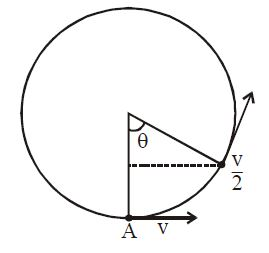# Vertical Circular Motion!A bob of mass $M$ is suspended by mass-less string of length $L$. The horizontal velocity at point $A$ is such that the tension at point $A$ is five times the weight of bob. The angle '$\theta$' at which the speed of bob is half of speed at point $A$ is:

×